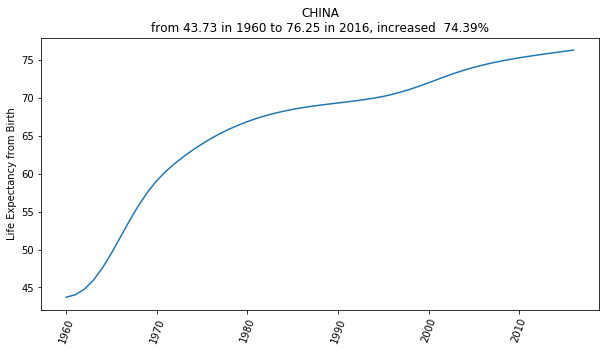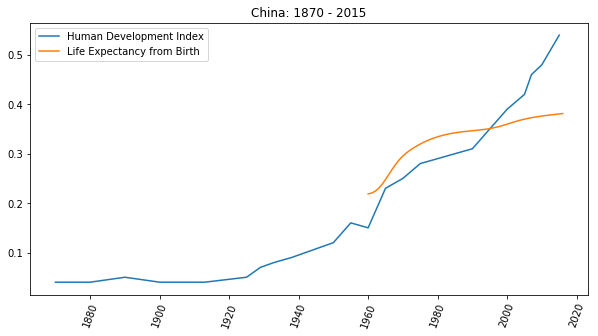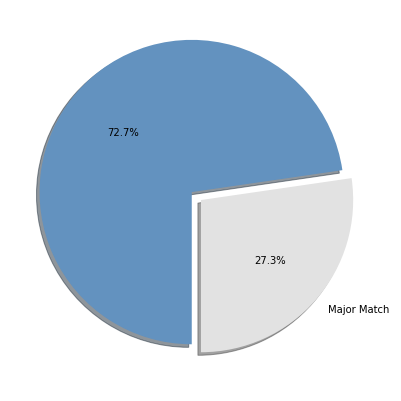Article Outline

为什么一定要掌握自学能力？

• 未来的日子还很长
• 这世界进步得太快

import matplotlib.pyplot as plt
import numpy as np

data = np.genfromtxt('life-expectancy-china-1960-2016.txt',
delimiter=',',
names=['x', 'y'])
da1960  = data
da2016  = data[-1]
increase = (da2016 - da1960) / da1960
note = 'from {:.2f} in 1960 to {:.2f} in 2016, increased  {:.2%}'\
.format(da1960, da2016, increase)

plt.figure(figsize=(10, 5))
plt.plot(data['x'], data['y'])
plt.ylabel('Life Expectancy from Birth')
plt.tick_params(axis='x', rotation=70)
plt.title('CHINA\n' + note)

# plt.savefig('life-expectancy-china-1960-2016.png', transparent=True)
plt.show()

# data from:
# https://databank.worldbank.org/data/reports.aspx?source=2&series=SP.DYN.LE00.INimport matplotlib.pyplot as plt
import numpy as np
plt.figure(figsize=(10, 5))

lebdata = np.genfromtxt('life-expectancy-china-1960-2016.txt',
delimiter=',',
names=['x', 'y'])

hdidata = np.genfromtxt('hdi-china-1870-2015.txt',
delimiter=',',
names=['x', 'y'])

plt.plot(hdidata['x'], hdidata['y'], label='Human Development Index')
plt.tick_params(axis='x', rotation=70)
plt.title('China: 1870 - 2015')

plt.plot(lebdata['x'], lebdata['y'] * 0.005, label='Life Expectancy from Birth')
plt.plot(secondary_y=True)

plt.legend()

# plt.savefig('human-development-index-china-1870-2015.png', transparent=True)
plt.show()

# https://ourworldindata.org/human-development-index

# data from:
# blob:https://ourworldindata.org/44b6da71-f79e-42ab-ab37-871e4bd256e9import matplotlib.pyplot as plt

labels = ['Major Match', '']
sizes = [273, 727]
colors = ['#E2E2E2', '#6392BF']
explode = (0, 0.08)
plt.figure(figsize=(7, 7))
plt.pie(sizes,
labels=labels,
explode=explode,
autopct='%1.1f%%',
colors=colors,
startangle=270,
# plt.savefig('major-match-job.png', transparent=True)
plt.show()

# data from:“你回头看看呗，想想呗，五年前你在做什么？是不是回头一看的时候，五年前就好像是昨天？道理是一样的，五年之后的某一天你回头想今天，也是 ‘一转眼五年就过去’ 了…… 只不过，你今天觉得需要时间太多，所以不肯学 —— 但是，不管你学还是不学，五年还是会 ‘一转眼就过去’ 的…… 到时候再回头，想起这事的时候，没学的你，一定会后悔 —— 事实上，你已经有很多次后悔过 ‘之前要是学了就好了’，不是吗？”

—— 我希望你也有。